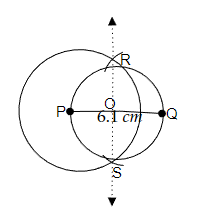# With $\overline{\mathrm{PQ}}$ of length $6.1 \mathrm{~cm}$ as diameter, draw a circle.

To do:

We have to draw a circle with a length $6.1\ cm$ as its diameter.

Solution:Steps of construction:

(i) Let us draw $\overline{PQ}$ of length $6.1\ cm$.

(ii) By taking a measure greater than half of the length of $\overline{PQ}$ and pointing the pointer of the compasses on the point P let us draw a circle.

(iii) With the same measure by pointing the pointer of the compasses at point Q let us draw two arcs on the formed circle and point them as R and S.

(iv) Now, let us join the points R and S. This intersects with $\overline{PQ}$ at a point let us name this intersection as O.

(v) Now,  by pointing the pointer of compasses at point O with a measure of the radius from point P to O let us draw another circle passing through the point Q.

(vi) Therefore, the required circle with a diameter $6.1\ cm$ is formed.

Updated on: 10-Oct-2022

24 Views